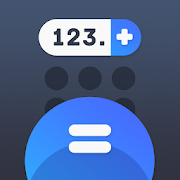# Calculator Plus - All-in-one Multi Calculator Free

## The Best Scientific Calculator with Currency, Unit Converter & Math tools Free!⚡

4.37 PRODUCTIVITY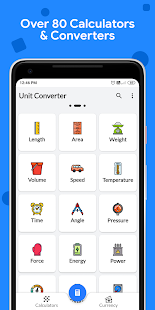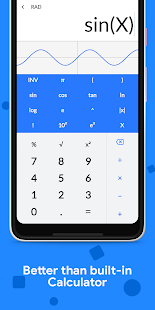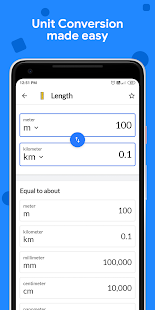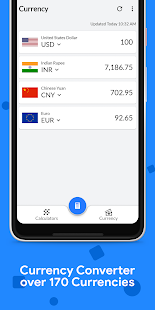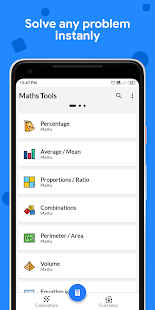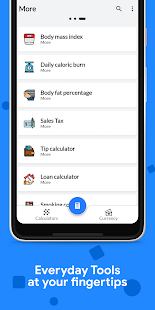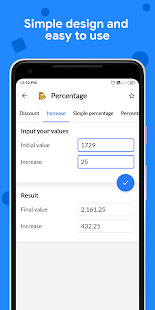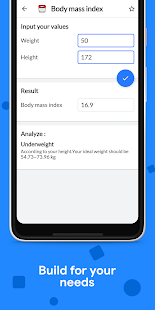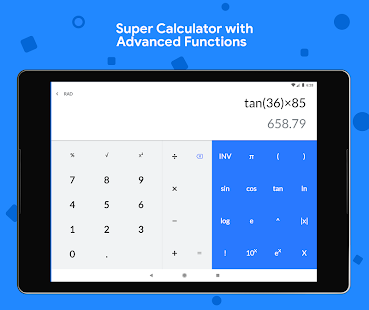Calculator Plus is a complete and easy to use multi calculator app which allows you to handle all necessary calculations for everyday life. A perfect💯 calculating tool specially built and beautifully designed for you.
⚡It is absolutely FREE!⚡

This all in one calculator app is the best utility 💡 which helps you to solve daily simple calculations and problems from complex calculations to the unit and currency conversions percentage equations areas volumes BMI loan tax and so on. Calculator Plus is the only calculator you will ever need on your device.

Key Features
• Standard calculator Simple or Scientific layout 🌈
• Convert units or currencies in the same app
• Over 80 calculators and unit converters
• Currency converter 💸 with 170 currencies (available offline)
• Solves your school homework 💡 instantly
• Calculator with Function graphing 📈 & History
• Smart search for faster navigation
• 🌃 Dark theme for late-night sessions
• Easy and quick navigation across app

It is a perfect tool for students teachers engineers handyman contractor or just somebody who struggles with math & conversions. Featuring over 80 free calculators and unit converters fully packed in with a scientific calculator and much better than your phone or handheld calculator.😎

The whole package helps you to solves any simple problem or advanced calculation instantly and accurately. The scientific calculator is equipped with function graphing calculation history advanced math functions and editable input. Its material design with clean interface allows you to focus on your calculations and data even in late-night sessions.💯✨

Math Tools
• Percentage calculator
• Average calculator - arithmetic geometric and harmonic means
• Proportion calculator
• Combinations and permutations
• Area / Perimeter calculator for triangle square rectangle parallelogram trapezoid rhombus pentagon hexagon circle circle arc and ellipse
• Volume calculator for cube rect. prism sq. pyramid sq. pyramid frustum cylinder cone conical frustum sphere spherical cap spherical segment and ellipsoid
• Equation solver - linear quadratic and equation system
• Decimal to fraction
• Prime number checker
• Right triangle calculator
• Heron’s formula (solve a triangle knowing the side lengths)
• Circle solver
• GCF and LCM calculator
• Fraction simplifier
• Number base converter
• Random number generator

Unit converters
• Length converter
• Area converter
• Weight converter
• Volume converter
• Speed converter
• Temperature converter
• Time converter
• Angle converter
• Pressure converter
• Force converter
• Energy converter
• Power converter
• Acceleration converter
• Torque converter
• Digital storage converter
• Data rate converter
• Prefix converter
• Image converter
• Charge converter
• Current converter
• Resistance converter
• Conductance converter
• Inductance converter
• Capacitance converter
• Fuel economy converter
• Cooking converter
• Frequency converter
• Illuminance converter
• Radiation converter

More
• Body mass index - BMI calculator
• Daily calories burn
• Body fat percentage calculator
• Sales Tax calculator
• Tip calculator
• EMI / Loan calculator
• Smoking cost calculator
• Age calculator
• Elapsed time calculator - years & days hours & minutes calculator

📧 Contact us at email feedbackgigantic@gmail.com We look forward to your feedback.

### About this version (2.1.1)

• UI enhancements • Bug fixes and performance improvements

### Other versions

2.1.0 released on 12 April 2020 (375 days ago)

### Details and Recent Ratings

Size: 5 MB
Version: 2.1.1 by Gigantic Apps
Updated: 08 June 2020 (318 days ago)
Released: 10 June 2018
Installations: more than 10 000
5 Stars: 216
4 Stars: 40
3 Stars: 21
2 Stars: 12
1 Star: 18

### Similar apps

Android Game Categories
Android App Categories